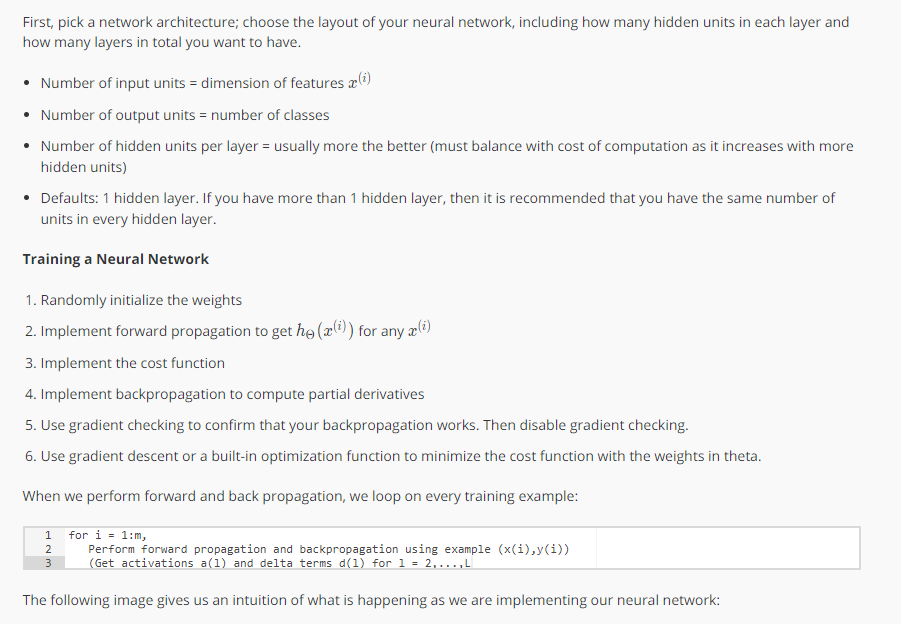## 直观感受反向传播的概念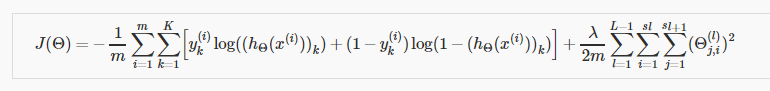\delta_j^(l) = \frac{\partial}{\partial z_j^(l)}cost(t)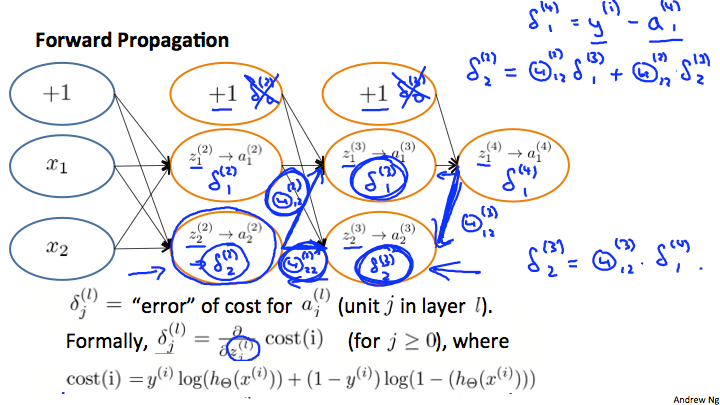\delta_2^(2) = \Theta_{12}^(2)\delta_1^(3) + \Theta_{12}^(2)\delta_1^(3)

\delta_2^(3) = \Theta_{12}^(3)\delta_1^(4)

## 参数展开

thetaVector = [Theta1(:);Theta2(:),Theta(3);]

Theta1 = reshape(thetaVector(1:110),10_11) \
Theta2 = reshape(thetaVector(111:220),10_11) \
Theta3 = reshape(thetaVector(221:231),1*11) \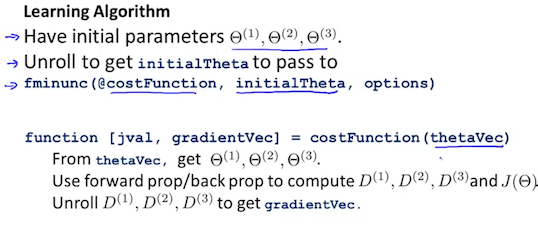## 梯度校验

\frac{\partial}{\partial \Theta}J(\Theta) \approx \frac{J(\Theta+\epsilon) – J(\Theta – \epsilon)}{2\epsilon}

\frac{\partial}{\partial \Theta}J(\Theta) \approx \frac{J(\Theta_1,… \Theta_j+\epsilon,…,\Theta_n) – J(\Theta_1…,\Theta_j – \epsilon,… \Theta_n)}{2\epsilon}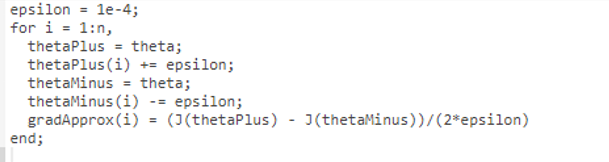## 随机初始化参数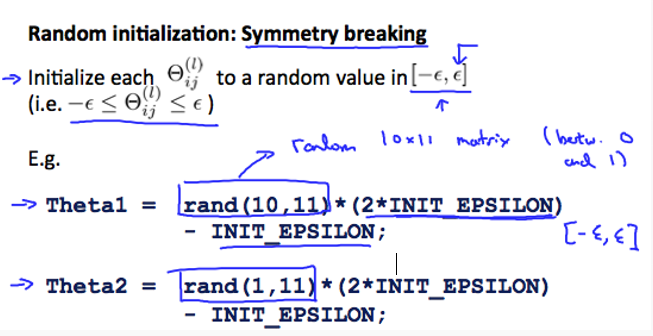Theta1 = rand(10,11) * (2*INIT_EPSILON) - INIT_EPSILON;
Theta2 = rand(10,11) * (2*INIT_EPSILON) - INIT_EPSILON;
Theta3 = rand(1,11) * (2*INIT_EPSILON) - INIT_EPSILON;


## 总结